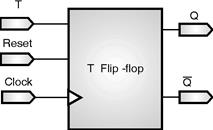Home > VHDL > Flipflops > TFFToggle Flip-Flops :

* To infer toggle flip-flops, you must include asynchronous controls in the toggle flip-flop description. Without asynchronous controls, you cannot initialize toggle flip-flops to a known state.

* Following example provides the VHDL code for a toggle flip-flop with asynchronous reset. Figure shows the inferred flip-flop.

library ieee;

use ieee.std_logic_1164.all;

entity T_FF is

port (T, Clock, Reset : in std_ulogic;

Q, Qbar : out std_ulogic);

end T_FF;

architecture var of T_FF is

begin

process (Clock, Reset) is

variable state : std_ulogic;

begin

if (Reset = '0') then

state := '0';

elsif rising_edge(Clock) then

if T = '1' then

state := not state;

end if ;

end if ;

Q <= state;

Qbar <= not state;

end process;

end var;